# How to Calculate and Solve for Free Air Anomaly | Gravity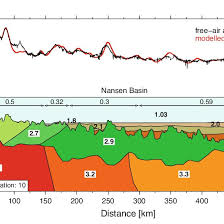The image above represents free air anomaly.

To compute for free air anomaly, four essential parameters are needed and these parameters are Observed or Measured Gravity Value (gobs), Reference Value of Gravity (g(λ)), Elevation (h) and Radius of the Earth (R).

The formula for calculating free air anomaly:

δgF = gobs – g(λ) [1 – 2h/R]

Where:
δgF = Free Air Anomaly
gobs = Observed or Measured Gravity Value
g(λ) = Reference Value of Gravity
h = Elevation
R = Radius of the Earth

Let’s solve an example;
Find the free air anomaly when the observed or measured gravity value is 9, reference value of gravity is 10, elevation is 16 and radius of the earth is 4.

This implies that;

gobs = Observed or Measured Gravity Value = 9
g(λ) = Reference Value of Gravity = 10
h = Elevation = 16
R = Radius of the Earth = 4

δgF = gobs – g(λ) [1 – 2h/R]
δgF = 9 – 10 [1 – 2(16)/4]
δgF = 9 – 10 [1 – 32/4]
δgF = 9 – 10 [1 – 8]
δgF = 9 – 10 [-7]
δgF = 9 – -70
δgF = 79

Therefore, the free air anomaly is 79 Gal.

Nickzom Calculator – The Calculator Encyclopedia is capable of calculating the free air anomaly.

To get the answer and workings of the free air anomaly using the Nickzom Calculator – The Calculator Encyclopedia. First, you need to obtain the app.

You can get this app via any of these means:

To get access to the professional version via web, you need to register and subscribe for NGN 2,000 per annum to have utter access to all functionalities.
You can also try the demo version via https://www.nickzom.org/calculator

Apple (Paid) – https://itunes.apple.com/us/app/nickzom-calculator/id1331162702?mt=8
Once, you have obtained the calculator encyclopedia app, proceed to the Calculator Map, then click on Geology under Add-on.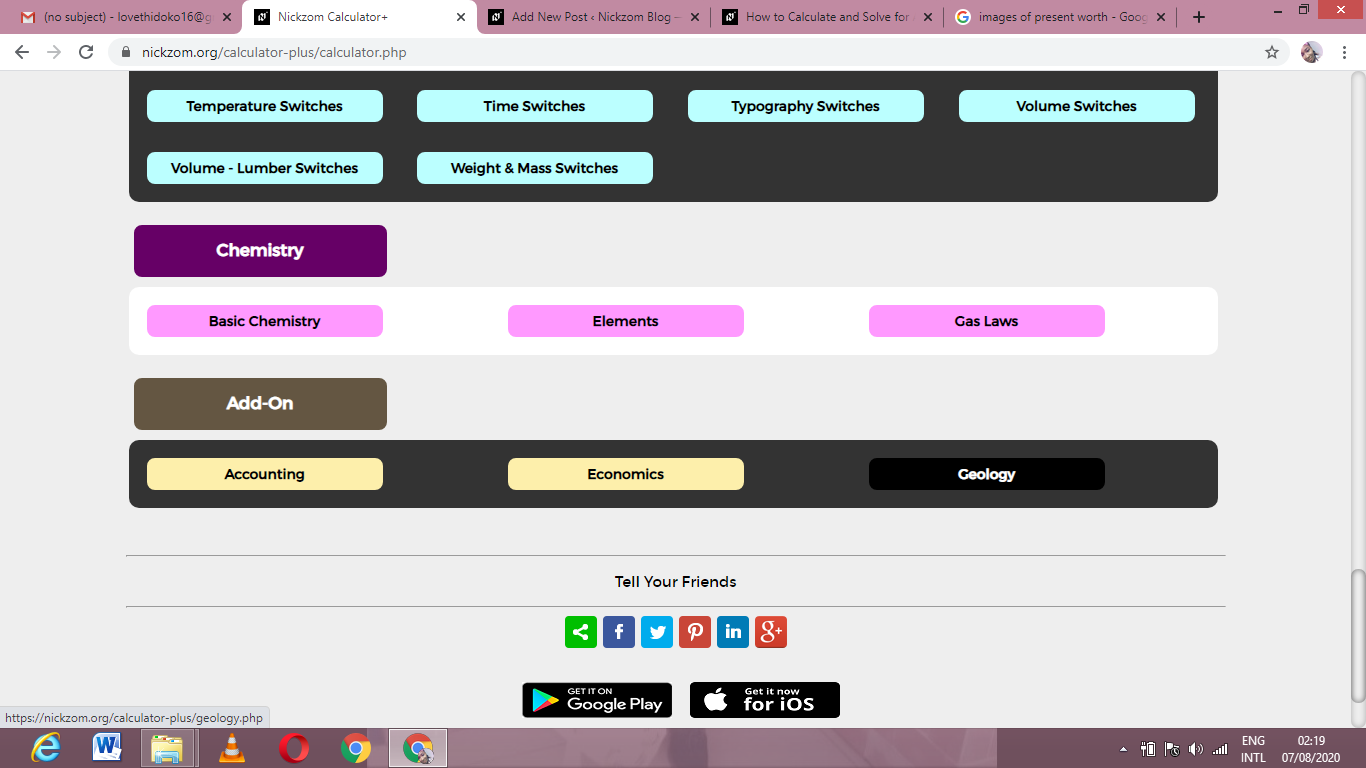Now, Click on Gravity under Geology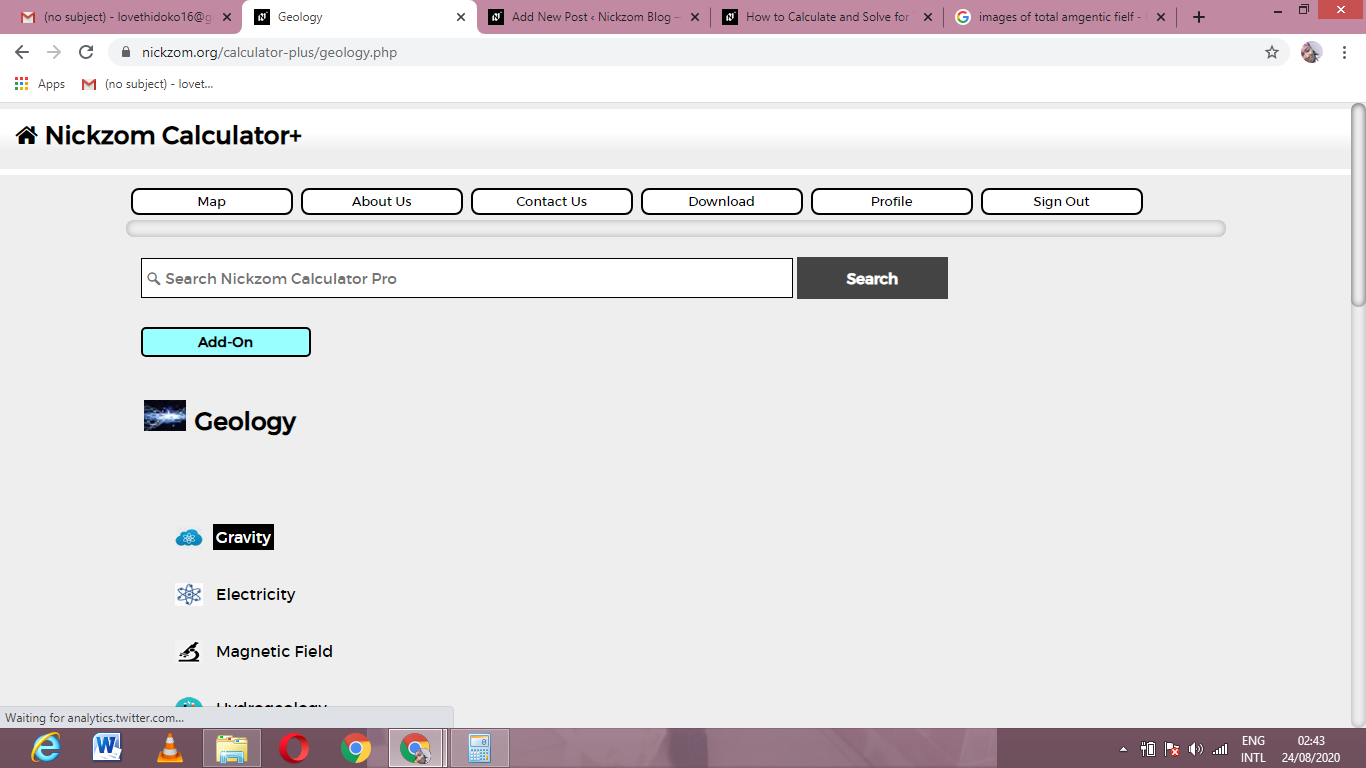Now, Click on Free Air Anomaly under Gravity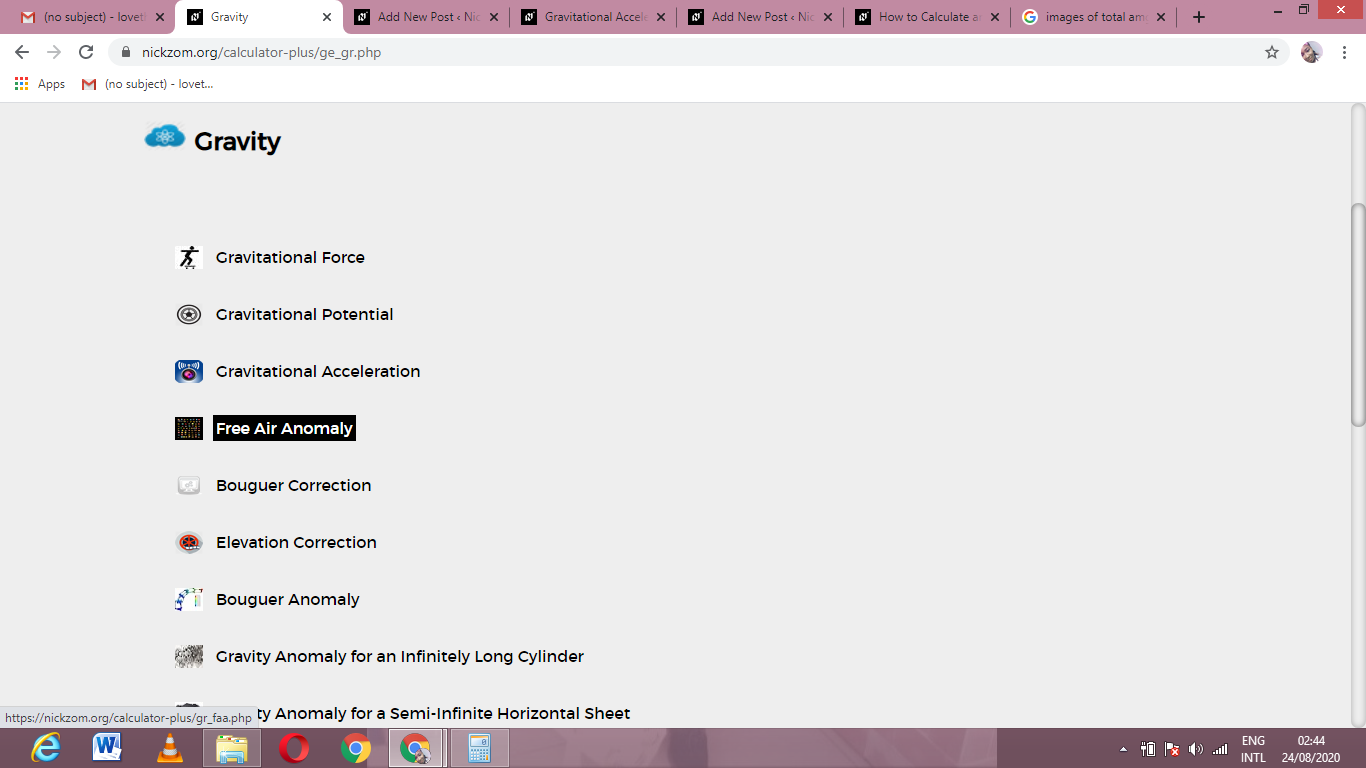The screenshot below displays the page or activity to enter your values, to get the answer for the free air anomaly according to the respective parameters which are the Observed or Measured Gravity Value (gobs), Reference Value of Gravity (g(λ)), Elevation (h) and Radius of the Earth (R).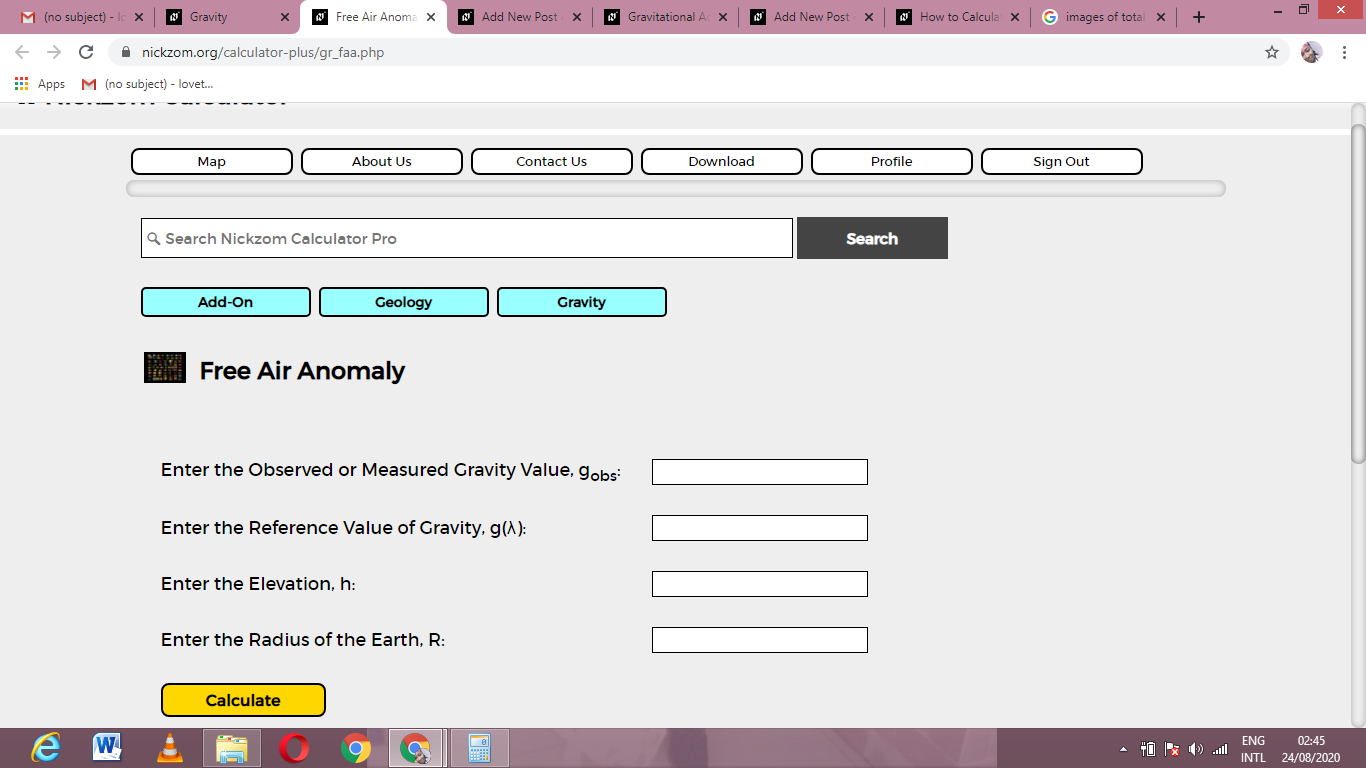Now, enter the values appropriately and accordingly for the parameters as required by the Observed or Measured Gravity Value (gobs) is 9, Reference Value of Gravity (g(λ)) is 10, Elevation (h) is 16 and Radius of the Earth (R) is 4.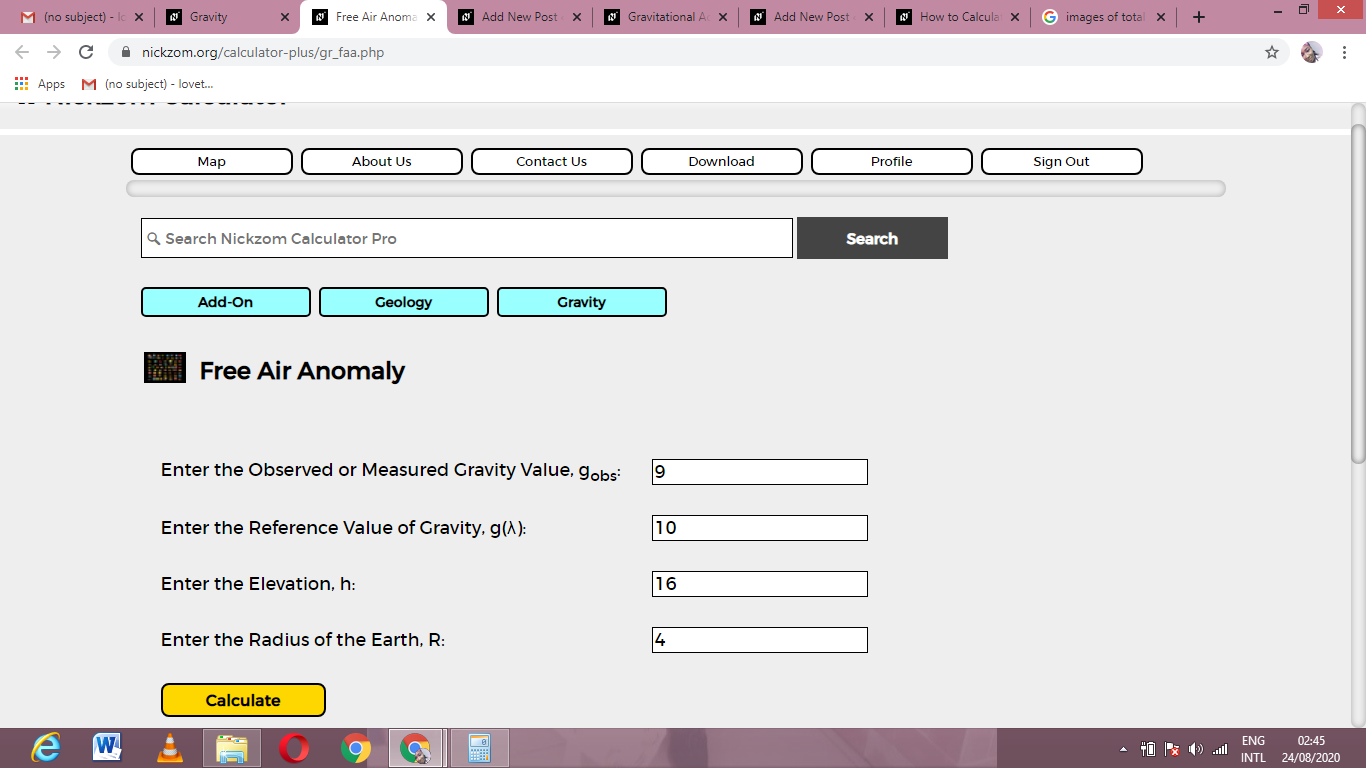Finally, Click on Calculate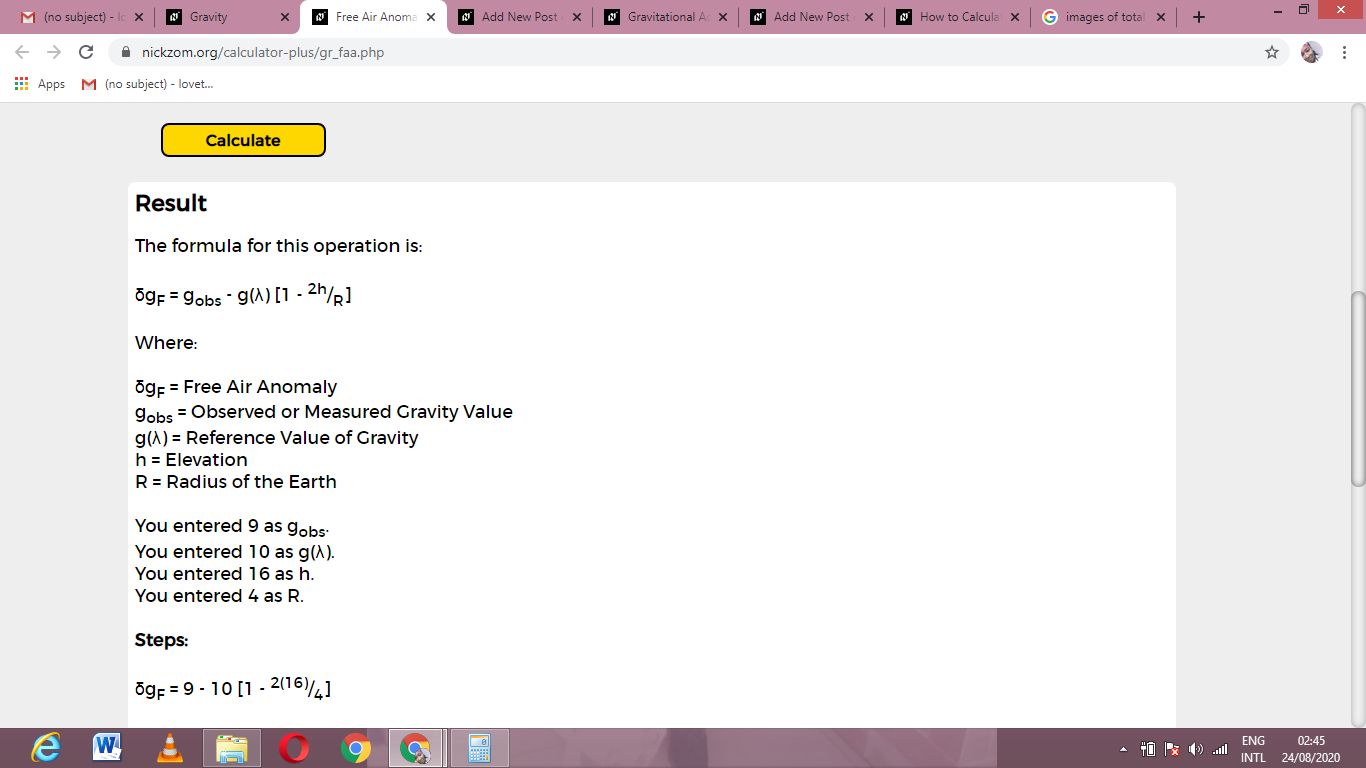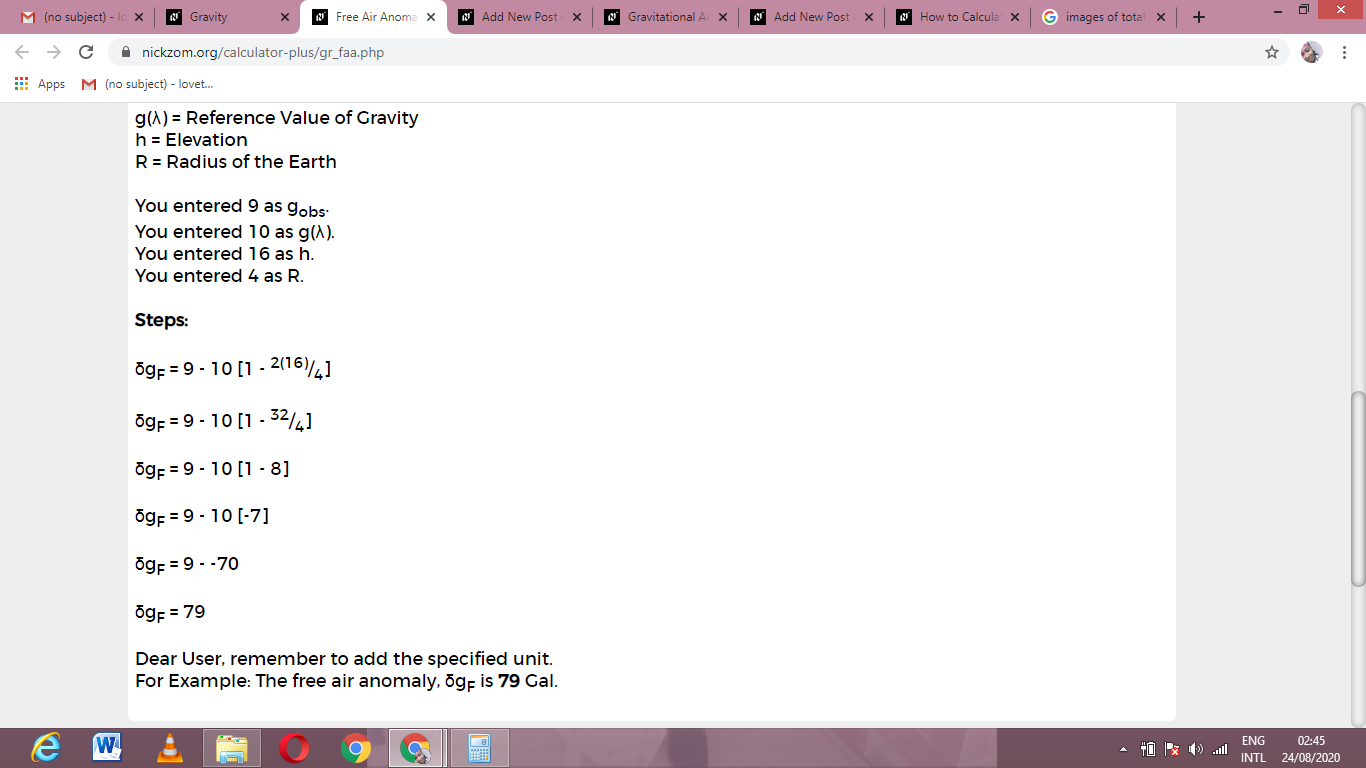As you can see from the screenshot above, Nickzom Calculator– The Calculator Encyclopedia solves for the free air anomaly and presents the formula, workings and steps too.